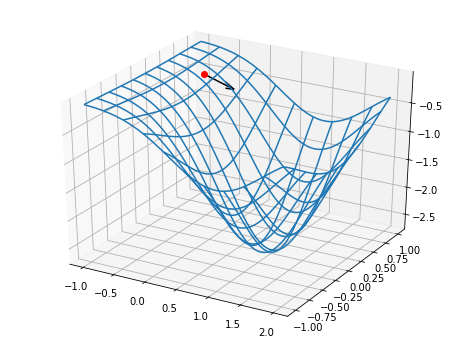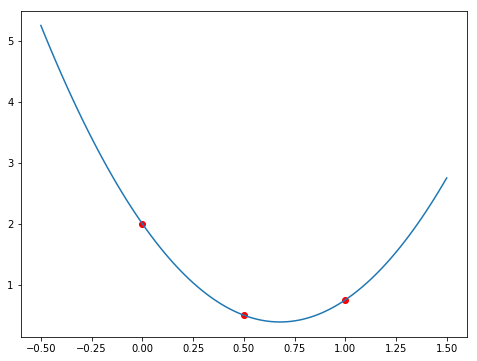In :
%matplotlib notebook
from mpl_toolkits.mplot3d import axes3d
import matplotlib.pyplot as plt


# Method of Steepest Descent¶

We use the method of Steepest Descent to find the coordinates $\vec{x} = (x_1, x_2, ... x_n)$ that minimizes the function

$$g: \mathbb{R}^n \rightarrow \mathbb{R}$$

To do this, we use the gradient of the function:

$$\nabla g(\vec{x}) = (\frac{\partial g}{\partial x_1}, \frac{\partial g}{\partial x_2}, ... , \frac{\partial g}{\partial x_n})$$

which has two interesting properties for our purposes. Much like the derivative of a function of 1 variable, the gradient is zero when $g$ is at a maximum or a minimum. Also, the directional derivative of $g$ at $\vec{x}$ in a given direction, specified by the unit vector $\vec{v}$ is given by

$$D_v g(\vec{x}) = \lim_{h \rightarrow 0} \frac{1}{h} [g(\vec{x} + h\vec{v}) - g(\vec{x})] = \vec{v}^t \cdot \nabla g(\vec{x})$$

Clearly if $\vec{v}$ is chosen to be in the direction of $\nabla g$, the change in $g$ is maximized. Therefore if we want to move towards the minimum of $g$ step by step, we should move in the direction of $-\nabla g$.

Assume we start from a point (the red dot on the graph below), our first approximation of where the minimum is, $\vec{x}^{(0)}$. To find the next point (the black arrow), $\vec{x}^{(1)}$, we move in the opposite direction of the gradient vector, multiplied by an amount that is proportional to $\alpha$.

$$\vec{x}^{(1)} = \vec{x}^{(0)} - \alpha \nabla g (\vec{x}^{(0)})$$

To find a proper value for $\alpha$, we can construct another function

$$h(\alpha) = g(\vec{x}^{(0)} - \alpha \nabla g(\vec{x}^{(0)}))$$

and minimize it by taking its derivative. This, in general, is very costly. What we do instead is, we choose 3 numbers $\alpha_1 < \alpha_2 < \alpha_3$, which we hope are close to the minimum of $h$ and form a quadratic polynomial $P(\alpha)$ that interpolates at $\alpha_1$, $\alpha_2$, and $\alpha_3$.

For example, we choose $\alpha_1 = 0$. Then we find $\alpha_3$ such that $h(\alpha_3) < h(\alpha_1)$. Start by setting $\alpha_3 = 1$. Then we set $\alpha_2 = \alpha_3 / 2$. We find the polynomial $P(\alpha)$ that passes through these three points. The minimum of $P(\alpha)$ in the interval $[\alpha_1, \alpha_3]$, called it $\hat{\alpha}$, occures either at the only critical point of $P$ or at the end-point $P(\alpha_3)$, since $P(\alpha_3) = h(\alpha_3) < h(\alpha_1) = P(\alpha_1)$. We then move to the point

$$\vec{x}^{(1)} = \vec{x}^{(0)} - \hat{\alpha} \nabla g (\vec{x}^{(0)})$$

Note: A straight-forward way to find the interpolation $P(\alpha)$ between points, 0, $\alpha_2$, and $\alpha_3$ is Newton's forward divded-difference formula (which we didn't talk about in the class).

$$P(\alpha) = g_1 + h_1 \alpha + h_3 \alpha (\alpha - \alpha_2)$$

where

$$g_1 = g(\vec{x}^{(0)}) \\ g_2 = g(\vec{x}^{(0)} - \alpha_2 \vec{z}) \\ g_3 = g(\vec{x}^{(0)} - \alpha_3 \vec{z}) \\ \vec{z} = \nabla g(\vec{x}^{(0)}) \\ h_1 = \frac{g_2 - g_1}{\alpha_2} \\ h_2 = \frac{g_3 - g_2}{\alpha_3 - \alpha_2} \\ h_3 = \frac{h_2 - h_1}{\alpha_3} \\$$

In :
g = lambda x,y: -1*np.exp(-(x**2 + y**2 -2*x))
a = g(x,y)
dgdx = (-2*x+2)*a
dgdy = -2*y*a
return np.array([dgdx, dgdy])

x0 = [-0.5, 0.5]

fig = plt.figure(figsize=(8,6))

Nx, Ny = (100, 100)
X, Y = np.meshgrid(np.linspace(-1, 2, Nx), np.linspace(-1, 1, Ny))
Z = g(X,Y)

# Plot a basic wireframe.
ax.plot_wireframe(X, Y, Z, rstride=10, cstride=10)
ax.plot([x0], [x0], [g(x0, x0)], 'or')
ax.quiver([x0], [x0], [g(x0, x0)], [v0], [v0], , color='k')

Out:
<mpl_toolkits.mplot3d.art3d.Line3DCollection at 0x7fc2c8948390>Here is an example to demonstrate how to find a second degree polynomial $P(x)$ that passes through three points $(0, g_1)$, $(a_2, g_2)$, and $(a_3, g_3)$.

In :
a1 = 0.
a2 = 0.5
a3 = 1.
g1 = 2.
g2 = 0.5
g3 = 0.75
h1 = (g2 - g1) / a2
h2 = (g3 - g2) / (a3 - a2)
h3 = (h2 - h1) / a3
points = np.array([[a1, g1], [a2, g2], [a3, g3]])
P = lambda x: g1 + h1 * x + h3 * x * (x - a2)

x = np.linspace(-0.5, 1.5, 100)
y = P(x)

fig = plt.figure(figsize=(8,6))

[<matplotlib.lines.Line2D at 0x7fc2c87dc320>]